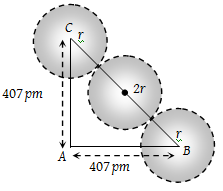# NEET Chemistry The Solid State Questions Solved

Silver metal crystallises in a cubic closest – packed arrangement with the edge of the unit cell having a length a = 407 pm. What is the radius of silver atom.

(1) 143.9 pm

(2) 15.6 pm

(3) 11.59 pm

(4) 13.61 pm

(1) $A{C}^{2}+A{B}^{2}=B{C}^{2}$here

${a}^{2}+{a}^{2}={\left(4r\right)}^{2}$

$2{a}^{2}=16{r}^{2}$

${r}^{2}=\frac{{a}^{2}}{8}$

$r=\frac{a}{2\sqrt{2}}=\frac{407}{2\sqrt{2}}=143.9\text{\hspace{0.17em}}pm$.

Difficulty Level:

• 71%
• 12%
• 11%
• 7%
Crack NEET with Online Course - Free Trial (Offer Valid Till August 28, 2019)